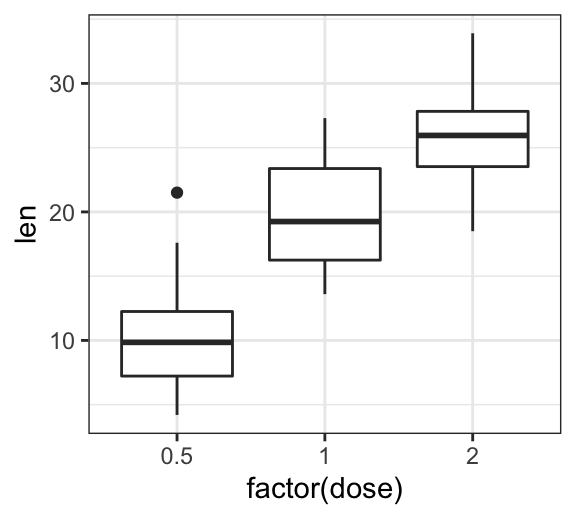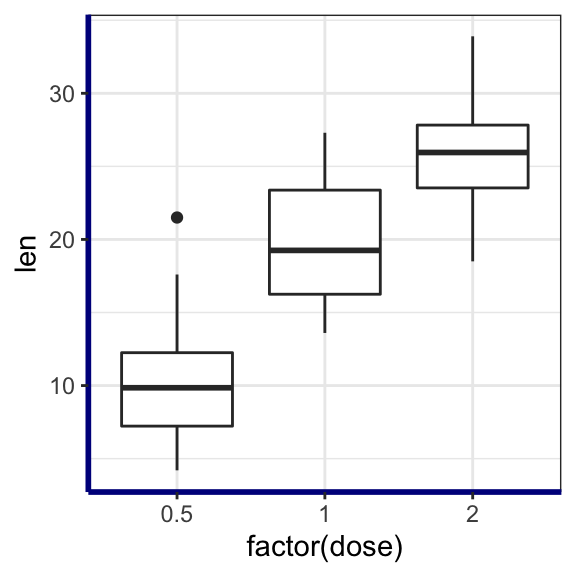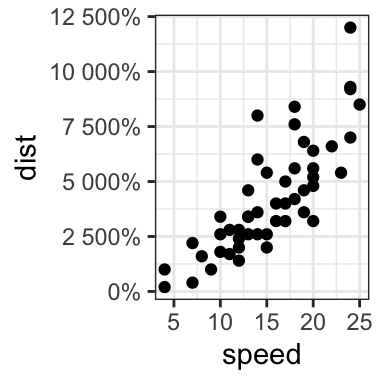# GGPlot Axis Ticks: Set and Rotate Text Labels#### GGPlot Axis Ticks: Set and Rotate Text Labels

This article describes how to easily set ggplot axis ticks for both x and y axes. We’ll also explain how to rotate axis labels by specifying a rotation angle.

In this R graphics tutorial, you will learn how to:

• Change the font style (size, color and face) of the axis tick mark labels.
• Rotate axis text labels. For example, for a vertical x axis text label you can specify the argument angle as follow: p + theme(axis.text.x = element_text(angle = 90)).
• Remove axis ticks mark and text: p + theme(axis.text.x = element_blank(), axis.ticks = element_blank()).
• Remove grid lines and customize axis lines.
• Customize axis ticks for discrete and continuous axes:
• Manually label / rename tick marks and change the order of items in the plot for a discrete x axis.
• Format continuous axis tick labels using percent, dollar and scientific scale transformations.
• Change the axis ticks interval (or breaks) by specifying custom values.

Contents:

## Key ggplot2 R functions

• Main functions:

The following function will be used to modify the axis theme and scale:

• theme(axis.text.x = element_text(), axis.text.y = element_text()). Change the appearance of axes text.
• theme(axis.line = element_line()). Change the axis lines
• scale_x_discrete() and scale_y_discrete(). Customize discrete x and y axes, respectively.
• scale_x_continuous() and scale_y_continuous(). Customize continuous x and y axes, respectively.

The scale functions take the arguments breaks, labels and limits as inputs.

• Key ggplot2 theme options to modify the axis line, axis ticks and tick text labels:
theme(
# Change axis lines
axis.line = element_line(),

# Change axis ticks text labels: font color, size and face
axis.text = element_text(),       # Change tick labels for all axes
axis.text.x = element_text(),     # Change x axis tick labels only
axis.text.x.top = element_text(), # x axis tick labels on top axis
axis.text.y = element_text(),     # Change y axis tick labels only
axis.text.y.right = element_text(),# y  axis tick labels on top axis

# Change axis ticks line: font color, size, linetype and length
axis.ticks = element_line(),      # Change ticks line fo all axes
axis.ticks.x = element_line(),    # Change x axis ticks only
axis.ticks.y = element_line(),    # Change y axis ticks only
axis.ticks.length = unit(3, "pt") # Change the length of tick marks
)

Arguments of the helper functions:

• element_text(color, size, face, family, angle, hjust, vjust). Modify the appearance and the rotation angle of axis texts.
• element_line(color, size, linetype). Modify the appearance of line elements.
• unit(). Change axis ticks length. Example: unit(4, “pt”).

To remove a particular axis text or ticks, use element_blank() for the corresponding theme argument. For example to remove all axis ticks, use this: p + theme(axis.ticks = element_blank()).

## Example of plots

Start by creating a box plot using the ToothGrowth data set:

library(ggplot2)
p <- ggplot(ToothGrowth, aes(x = factor(dose), y = len)) +
geom_boxplot()
p## Change axis tick mark labels

The functions theme() and element_text() are used to set the font size, color and face of axis tick mark labels. You can also specify the argument angle in the function element_text() to rotate the tick text.

Change the style and the orientation angle of axis tick labels. For a vertical rotation of x axis labels use angle = 90.

# Rotate x and y axis text by 45 degree
# face can be "plain", "italic", "bold" or "bold.italic"
p + theme(axis.text.x = element_text(face = "bold", color = "#993333",
size = 12, angle = 45),
axis.text.y = element_text(face = "bold", color = "blue",
size = 12, angle = 45))

# Vertical rotation of x axis text
p + theme(axis.text.x = element_text(angle = 90))To adjust the position of the axis text, you can specify the argument hjust and vjust, which values should be comprised between 0 and 1. For example:

# Create a new simple box plot
p2 <- ggplot(iris, aes(Species, Sepal.Length)) +
geom_boxplot()

# Rotated but not adjusted x axis text
p2 + theme(axis.text.x = element_text(angle = 45))

# Rotate and adjust x axis text
p2 + theme(axis.text.x = element_text(angle = 45, hjust = 1))## Remove x and y axis tick mark labels

Key function: element_blank()

# Remove x and y axis tick mark labels
p + theme(
axis.text.x = element_blank(),
axis.text.y = element_blank())

# Remove axis ticks and tick mark labels
p + theme(
axis.text.x = element_blank(),
axis.text.y = element_blank(),
axis.ticks = element_blank())## Change axis lines

• Key function: theme() and element_line()
• Allowed values for line types: (“blank”, “solid”, “dashed”, “dotted”, “dotdash”, “longdash”, “twodash”) or number (0, 1, 2, 3, 4, 5, 6). linetype = "solid" is identical to linetype = 1.

Change the color, the size and the line type of axis lines:

p + theme(
axis.line = element_line(color = "darkblue",
size = 1, linetype = "solid")
)## Customize continuous and discrete axes

x or y axis can be discrete (grouping variable) or continuous (numeric variable). In each of these two cases, the functions to be used for setting axis ticks are different.

Key ggplot2 R functions:

• Discrete axes:
• scale_x_discrete(name, breaks, labels, limits): for x axis
• scale_y_discrete(name, breaks, labels, limits): for y axis
• Continuous axes:
• scale_x_continuous(name, breaks, labels, limits, trans): for x axis
• scale_y_continuous(name, breaks, labels, limits, trans): for x axis

Function arguments definition:

• name: x or y axis labels
• breaks: vector specifying which breaks to display
• labels: labels of axis tick marks
• limits: vector indicating the data range

The scale_xx() functions can be used to change the following x or y axis parameters :

• axis titles or labels
• axis limits (data range to display)
• choose where tick marks appear
• manually label tick marks

### Discrete axes

In the examples below, we’ll use only the functions scale_x_discrete() and xlim() to customize x axis tick marks. The same type of examples can be applied to a discrete y axis using the functions scale_y_discrete() and ylim().

# Change x axis label and the order of items
p + scale_x_discrete(name ="Dose (mg)",
limits=c("2","1","0.5"))

# Rename / Change tick mark labels
p + scale_x_discrete(breaks=c("0.5","1","2"),
labels=c("D0.5", "D1", "D2"))

# Choose which items to display
p + scale_x_discrete(limits=c("0.5", "2"))

# or use this:
# p + xlim("0.5", "2") # same as above### Continuous axes

• Create a simple scatter plot
• Change axis labels and limits. Break axis by a user defined value.
# Simple scatter plot
sp <- ggplot(cars, aes(x = speed, y = dist)) + geom_point()
sp

# Change x and y axis labels, and limits
sp + scale_x_continuous(name="Speed of cars", limits=c(0, 30)) +
scale_y_continuous(name="Stopping distance", limits=c(0, 150))• Change axis ticks interval:
# Break y axis by a specified value
# a tick mark is shown on every 50
sp + scale_y_continuous(breaks=seq(0, 150, 50))

# Tick marks can be spaced randomly
sp + scale_y_continuous(breaks=c(0, 50, 65, 75, 150))• Remove tick mark labels and gridlines
• Format axis tick labels. Possible values for labels are comma, percent, dollar and scientific. For more examples, read the documentation of the function trans_new() in the scales R package.
# Remove y tick mark labels and grid lines
sp + scale_y_continuous(breaks=NULL)

# Format y axis labels in percent (%)
require(scales)
sp + scale_y_continuous(labels = percent)For dollar and scientific formats, type this:

require(scales)

# Dollar
p + scale_y_continuous(labels = dollar)

# Scientific
p + scale_y_continuous(labels = scientific)

## Conclusion

• Create an example of ggplot:
library(ggplot2)
p <- ggplot(iris, aes(Species, Sepal.Length)) +
geom_boxplot()
• Change tick font:
# Change tick text for both x and y axis
p + theme(
axis.text = element_text(size = 11, color = "blue")
)
# Change tick text for x axis ony
p + theme(axis.text.x = element_text(color = "blue", face = "italic"))
• Rotate x axis text:
# Vertical rotation: 90 degree
p + theme(
axis.text.x = element_text(angle = 90, hjust = 1, vjust = 0.5)
)
# Rotate by 45 degree
p + theme(
axis.text.x = element_text(angle = 45, hjust = 1)
)
• Remove x axis ticks and rename tick labels:
p + theme(axis.ticks.x = element_blank())+
scale_x_discrete(
breaks=c("setosa","versicolor","virginica"),
labels=c("SE", "VE", "VI")
)

•Truc

Thank you for this! Super helpful

•Kassambara

•Nas

Hi Kassambara,

•Kassambara

Thank you fr this positive feedback!

•Fernando Ascencio

Muchas gracias!!! // Thank you very much!!!

•Alejandro Hernández Cobos

Hi is posible combinate 2 codigos scale_X_discrete? maybe #Change and # remove?

•Lenny Lin

I am looking for a way of including texts to number ticks. For instance, instead of 7, I want to alternate it with “7 as pure water”, instead of 4, I want to alternate it with “4 like apple juice” on a pH scatter plot.